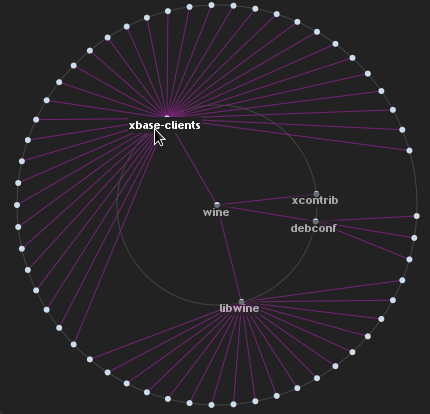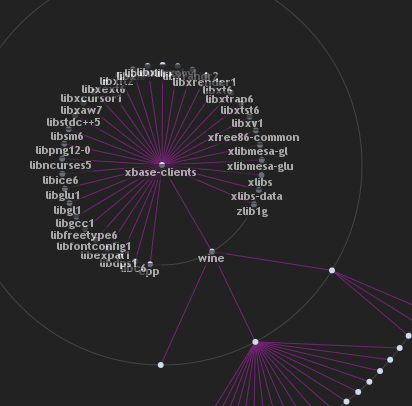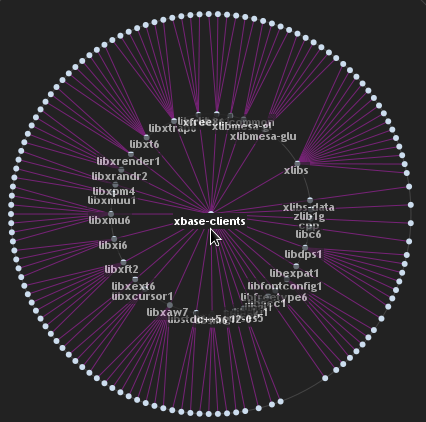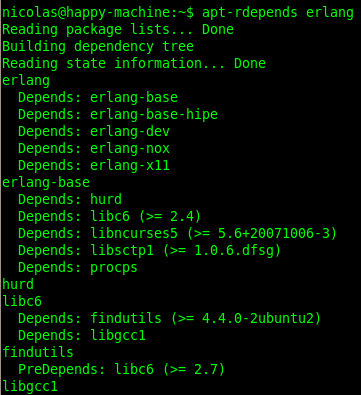# Visualizing Linux package dependencies

Posted in: javascript infovis toolkit , demo , tutorial
I've been building a Linux package dependency visualizer with Python and the JavaScript Infovis Toolkit that gathers all dependencies for a linux package and displays them in an interactive tree visualization. So, let's say your query is wine and you want to see dependencies for that package. The visualization will display wine as the centered node, laying its dependencies on outer concentric circles like this:By clicking on xbase-clients you'll set this node as root:Then, the visualization will query for xbase-clients dependencies, morphing its state into the new node's perspective:You can play with the example here. I'll explain how to build this in case you want your own at home. I guess this is going to be also a nice tutorial on how to configure the RGraph visualization to run advanced examples, including the new morphing animations in version 1.0.7a.

#### Server Side

Server side we need to build a service that can transform the apt-rdepends output for package dependencies into a JSON tree structure. The apt-rdepends is a linux tool (which you can install with apt-get install apt-rdepends) that displays a hierarchy of package dependencies for a given package. Here's an example when querying for erlang:You can either use popen2 or commands.getoutput to fetch the output for a system call in Python, I'll do the latter. The main function that makes the system call and returns the answer could be something like this:
``````def get_dependency_tree(package=''):
out = commands.getoutput("apt-rdepends " + package).split("\n")
ans = []
#if dependencies were found for this package.
if len(out) > 3 and out.strip() == package:
ans = out[3:]
else:
ans = [package]
return make_tree(package=ans.strip(), source=ans, level=2)``````
The make_tree function will create the tree structure that will then be serialized into JSON to be processed client side. We will first need a make_tree_node function that creates a tree node structure from a package's name:
``````#returns a tree node
def make_tree_node(id, node_name):
node_name = node_name.strip()
return {
'id': id,
'name': node_name,
'children': [],
'data': []
}``````
As you can see, this is the same tree node as the JSON tree structure defined for the JIT:
``````var json = {
"id": "aUniqueIdentifier",
"name": "usually a nodes name",
"data": [
{key:"some key",       value: "some value"},
{key:"some other key", value: "some other value"}
],
children: [/* other nodes or empty */]
};``````
Our make_tree function will receive as formal parameters the root package, the response from the apt-rdepends call, an integer that will specify the max depth for the tree (in case we want to prune it to some level) and an id prefix that will be set for each node:
``````def make_tree(package='', source=[], level=1, prefix=''):
node = make_tree_node(package + '_' + prefix, package)
if level > 0:
deps = get_package_deps(package, source)
[node['children'].append(make_tree(elem, source, level -1, package)) for elem in deps]
return node``````
As you can see, make_tree recursively creates nodes and appends them to their parent children property. Finally, I also made a get_package_deps function that retrieves all children for a given package, parsing source:
``````def get_package_deps(package_name='', source=[]):
ans, found_package_name = [], False
#test if is a dependency line
dependency = lambda package: package.strip().startswith('Depends:')
for line in source:
#package name line
found_package_name = True
elif found_package_name and dependency(line):
ans.append(line.split("Depends: ").split("(").strip())
#end of dependency lines
elif found_package_name and not dependency(line):
return ans
return ans``````
``````def apt_dependencies(request, mode, package):
json = aptdependencies.get_dependency_tree(package)
json_string = simplejson.dumps(json)
return render_to_response('raw.html', { 'json' : json_string })``````

#### Client Side

All the JavaScript Infovis Toolkit visualizations are customizable via controller methods. If this is the first time you use this library, perhaps it would be better to start with the RGraph quick tutorial first. First we define a simple Log object, that will write the current state of the graph to a label (like loading... or stuff like that). I'll use Mootools, but you can use whatever you want.
``````var Log = {
elem: false,
getElem: function() {
return this.elem? this.elem : this.elem = \$('log');
},

write: function(text) {
var elem = this.getElem();
elem.set('html', text);
}
};``````
Then we can define an init function, that instanciates the RGraph object and returns it. We will pass a controller to this object, that implements the onBeforeCompute, onAfterCompute, onPlaceLabel and onCreateLabel methods. I'll also define some utility methods, like requestGraph and preprocessTree:
``````function init() {
//Set node radius to 3 pixels.

//Create a canvas object.
var canvas= new Canvas('infovis', '#ccddee', '#772277');

//Instanciate the RGraph
var rgraph= new RGraph(canvas,  {
//Here will be stored the
//clicked node name and id
nodeId: "",
nodeName: "",

//Refresh the clicked node name
//and id values before computing
//an animation.
onBeforeCompute: function(node) {
Log.write("centering " + node.name + "...");
this.nodeId = node.id;
this.nodeName = node.name;
},

//Add a controller to assign the node's name
//and some extra events to the created label.
onCreateLabel: function(domElement, node) {
var d = \$(domElement);
'mouseenter': function() {
d.setOpacity(1);
},
'mouseleave': function() {
d.setOpacity(0.6);
},
'click': function() {
if(Log.elem.innerHTML == "done") rgraph.onClick(d.id);
}
});
},

//Once the label is placed we slightly
//change the positioning values in order
//to center or hide the label
onPlaceLabel: function(domElement, node) {
var d = \$(domElement);
d.setStyle('display', 'none');
if(node._depth &lt;= 1) {
d.set('html', node.name).setStyles({
'width': '',
'height': '',
'display':''
}).setStyle('left', (d.getStyle('left').toInt()
- domElement.offsetWidth / 2) + 'px');
}
},

//Once the node is centered we
//can request for the new dependency
//graph.
onAfterCompute: function() {
Log.write("done");
this.requestGraph();
},

//We make our call to the service in order
//to fetch the new dependency tree for
//this package.
requestGraph: function() {
var that = this, id = this.nodeId, name = this.nodeName;
Log.write("requesting info...");
var jsonRequest = new Request.JSON({
'url': '/service/apt-dependencies/tree/'
+ encodeURIComponent(name) + '/',

onSuccess: function(json) {
Log.write("morphing...");
//we preprocess the ids of the nodes
//in the graph and perform a morphing
//operation.
that.preprocessTree(json);
GraphOp.morph(rgraph, json, {
'id': id,
'duration':2000,
hideLabels:true,
onComplete: function() {
Log.write('done');
},
onAfterCompute: \$empty,
onBeforeCompute: \$empty
});
},

onFailure: function() {
Log.write("sorry, the request failed");
}
}).get();
},

//This method searches for nodes that already
//existed in the visualization and sets the new node's
//id to the previous one. That way, all existing nodes
//that exist also in the new data won't be deleted.
preprocessTree: function(json) {
var ch = json.children;
var getNode = function(nodeName) {
for(var i=0; i&lt;ch.length; i++) {
if(ch[i].name == nodeName) return ch[i];
}
return false;
};
json.id = rgraph.root;
var root = rgraph.graph.getNode(rgraph.root);
var nodeTo = elem.nodeTo, jsonNode = getNode(nodeTo.name);
if(jsonNode) jsonNode.id = nodeTo.id;
});
}

});

return rgraph;
}``````
I did say advanced example. You can always go to a simpler example to begin here. Finally we have to initialize the visualization when the page loads, so we'll attach an initialization function like this:
``````window.addEvent('domready', function() {
var rgraph = init();
new Request.JSON({
'url':'/service/apt-dependencies/tree/wine/',
onSuccess: function(json) {
//compute positions
rgraph.compute();
//make first plot
rgraph.plot();
Log.write("done");
rgraph.controller.nodeName = name;
},

onFailure: function() {
Log.write("failed!");
}
}).get();``````

#### HTML and CSS

These are the HTML and CSS files I used to make this example/tutorial. The HTML:
``````<!DOCTYPE html PUBLIC "-//W3C//DTD XHTML 1.0 Transitional//EN" "http://www.w3.org/TR/xhtml1/DTD/xhtml1-transitional.dtd">
<html xmlns="http://www.w3.org/1999/xhtml" xml:lang="en" lang="en">
<meta http-equiv="Content-Type" content="text/html; charset=UTF-8" />
<title>

Linux package dependency visualizer

</title>
<script type="text/javascript" src="/static/js/mootools-1.2.js"></script>

<!--[if IE]>
<script language="javascript" type="text/javascript" src="/static/js/excanvas.js"></script>
<![endif]-->
<script language="javascript" type="text/javascript" src="/static/js/core/RGraph.js"></script>
<script language="javascript" type="text/javascript" src="/static/js/example/example-rgraph.js"></script>

<canvas id="infovis" width="900" height="500"></canvas>
<div id="label_container"></div>

</body>
</html>
<div id="log"></div>``````
Note: You'll probably have to change the path to the CSS and JavaScript files. and the CSS file:
``````html,body {
width:100%;
height:100%;
background-color:#333;
text-align:center;
font-size:0.94em;
font-family:"Trebuchet MS",Verdana,sans-serif;
}

#infovis {
width:900px;
height:500px;
background-color:#222;

}

.node {
color: #fff;
background-color:#222;
font-weight:bold;